Courses
Courses for Kids
Free study material
Free LIVE classes
More

# What are Coordinates?## An Introduction to X and Y Coordinates

Have you ever thought about how you would represent a point (2,3)? This is done using a coordinate plane which is defined as a two-dimensional plane formed by the intersection of two lines, a vertical line called the y-axis and a horizontal line called the x-axis. These lines are perpendicular to each other, intersecting at a point called the origin. The given article covers all the concepts of coordinates, using attractive images to grab student's attention in learning. Students having a grip on this topic will be able to understand coordinate geometry easily.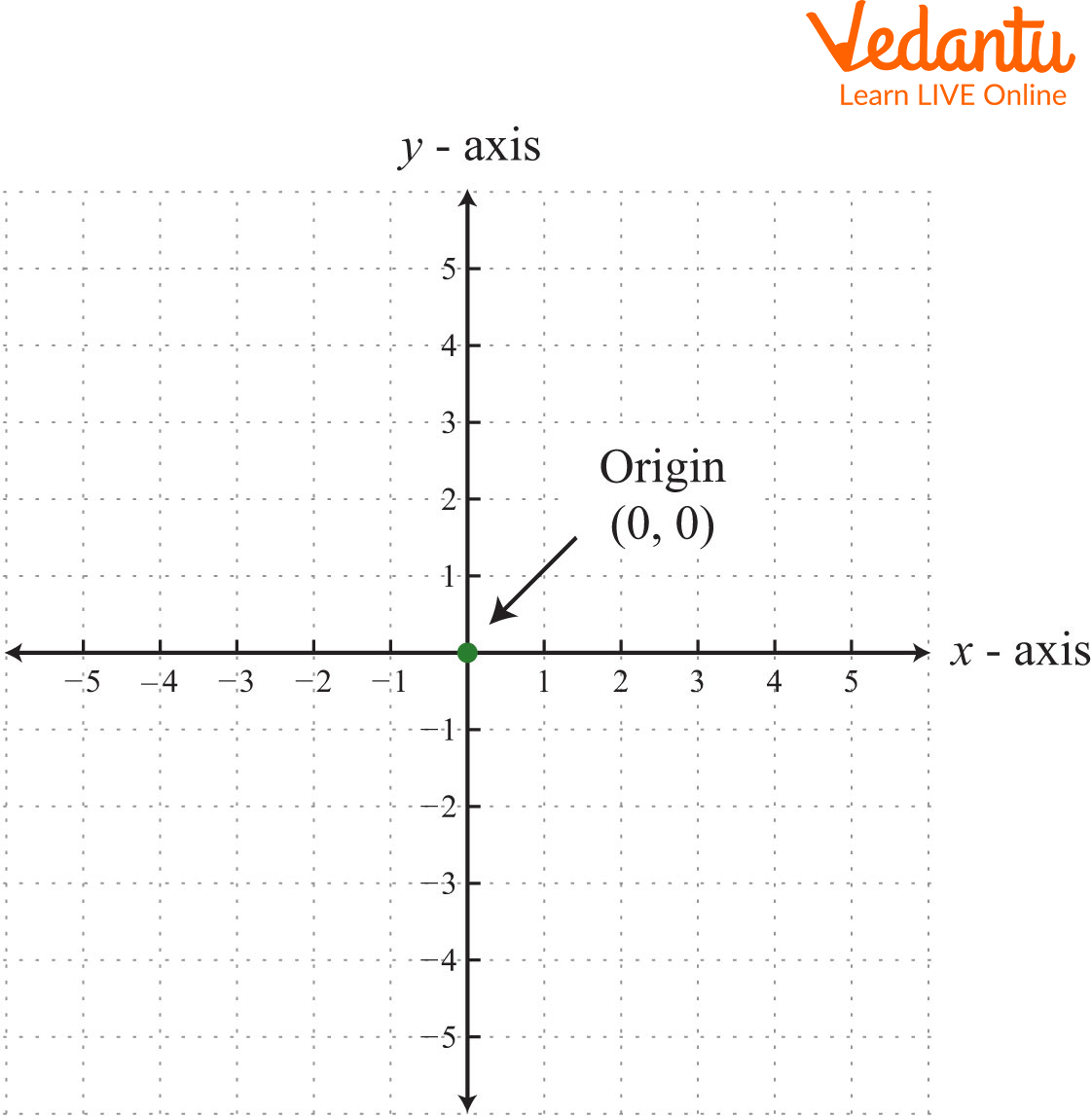Showing X and Y Coordinate Plane

## What are Coordinates?

Coordinates are a pair of numbers written in an ordered pair that identifies a specific point on a coordinate plane. Coordinates are represented using the ordered pair (x, y), which corresponds to the x and y coordinates, respectively. These coordinates can be positive, negative, or zero depending on the quadrant in which it lies.

## Grid for Coordinates

A coordinate grid is a two-dimensional surface, which represents a regular arrangement in which the horizontal lines of the x-axis intersect with the vertical lines of the y-axis to form regular blocks, resembling fishing nets. The point of the intersection of horizontal and vertical lines forms a right angle, i.e. an angle perpendicular to each other.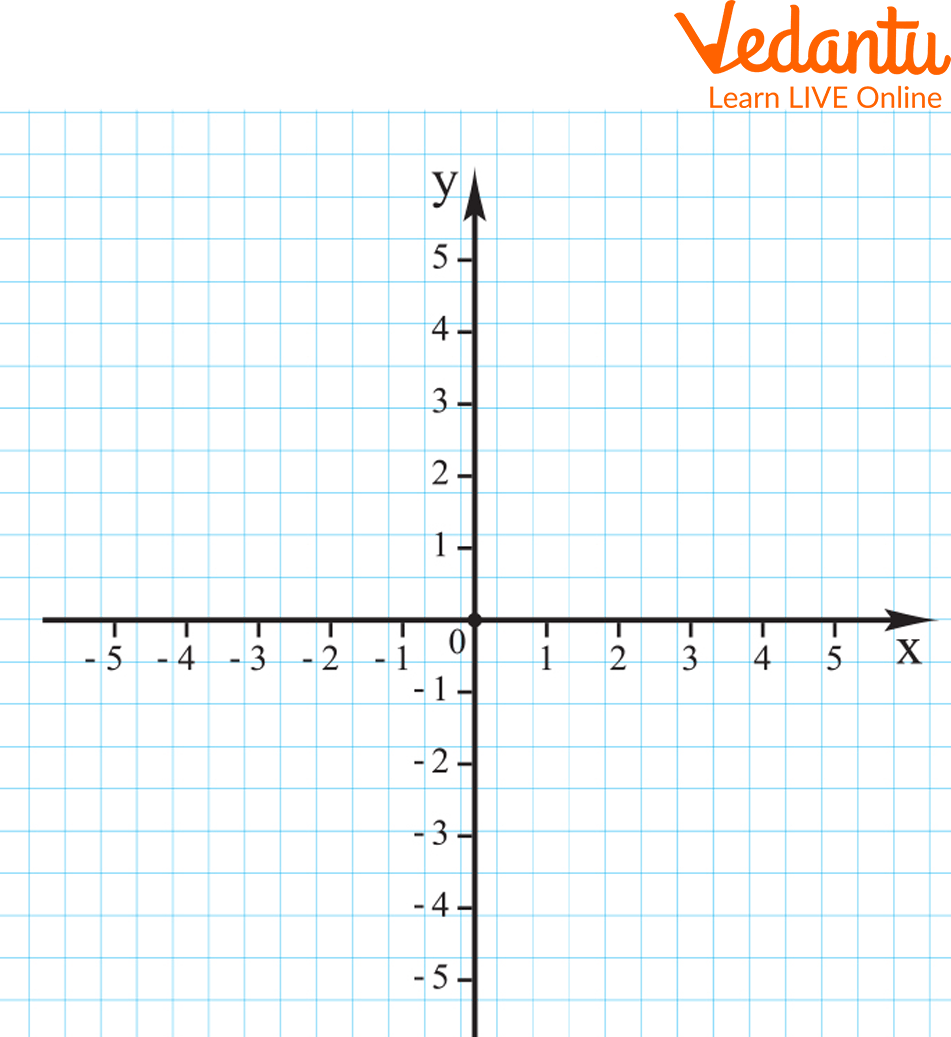Coordinates Grid

## Quadrants of the Grid for Coordinates

Quadrants are defined as that region of the plane which is gained after the intersection of the two axes. There are four quadrants in the grid for coordinates: namely,

Here, both the x and y coordinates are positive. For example: (2,3).

Here, the x coordinate is negative and the y coordinate is positive. For example: (-1,3).

Here, both the x and y coordinates are negative. For example: (-1,-5).

Here, the x coordinate is positive and the y coordinate is negative. For example: (6,-8).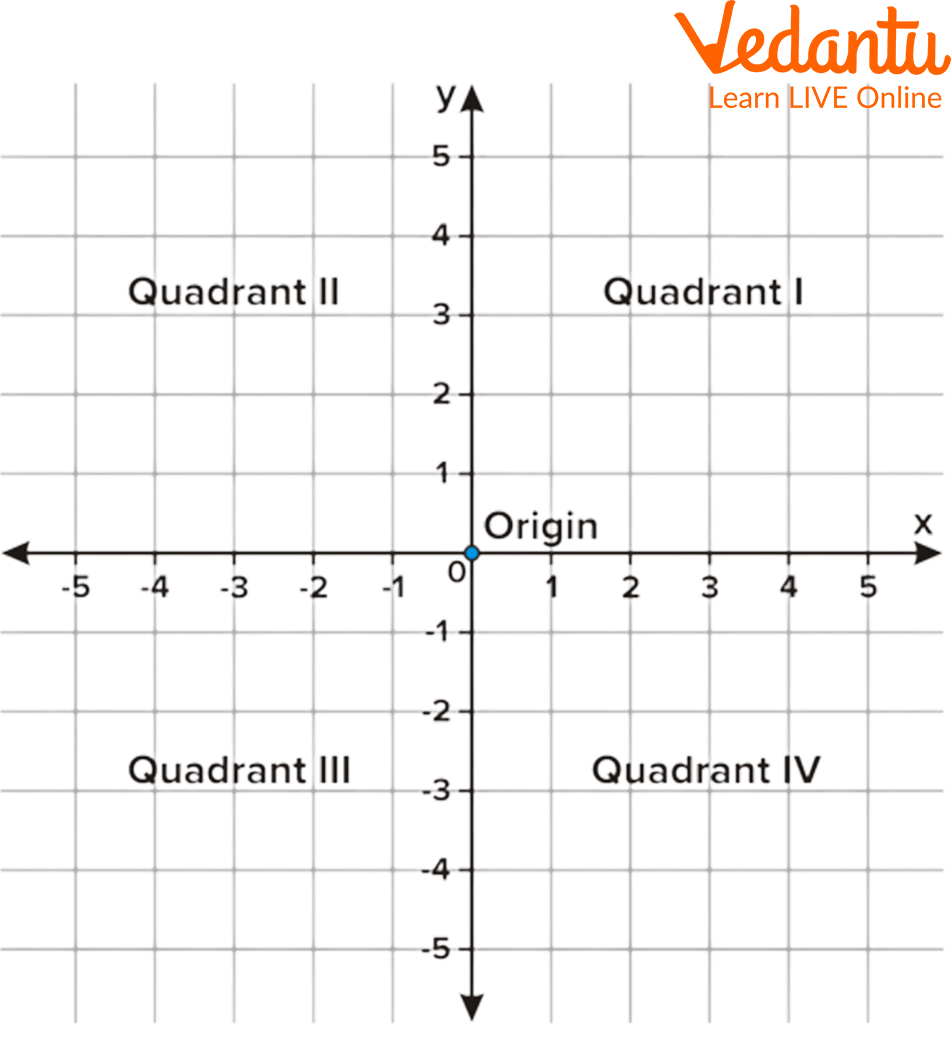Four Quadrants of a Grid of Coordinates

## How to Write Coordinates

Coordinates are written in an ordered form inside the brackets, separating each other with commas i.e. (x,y). The first term in the coordinate specifies the point on the x-axis and the second term specifies the point on the y-axis. Coordinate examples are: (3,4), (-3,-6), (6,-2), etc.

## Locating X and Y Coordinates

To locate xy coordinates on the graph, one needs to follow some steps:

1. Read the coordinate and find out in which quadrant it lies.

2. Mark the x and y coordinates in the respective quadrant.

3. Highlight the point and write the coordinates.

## Solved Coordinate Examples

Some coordinate examples are discussed underneath:

Q1. Plot (-3,2) on xy coordinates graph.

Ans: Using a simple series of steps to plot a point on the coordinate plane:

2. Mark the value of x=-3 and y=2 in the respective quadrant

3. Highlight the point and write its coordinates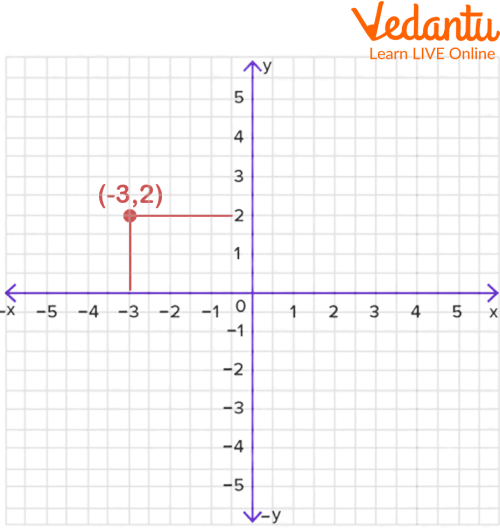Coordinates Images

Q2. Plot (4,2), (-3,4), (-4,0), (0,-5) on xy coordinates graph.

Ans: Using a simple series of steps to plot these points on the xy coordinate graph:

1. Read one of the given coordinates, say (4,2) and find out in which quadrant it lies, i.e. 1st quadrant.

2. Mark x=4 and y=2 in the respective quadrant.

3. Highlight the point and write its coordinate.

4. Repeat the above process for all other given points.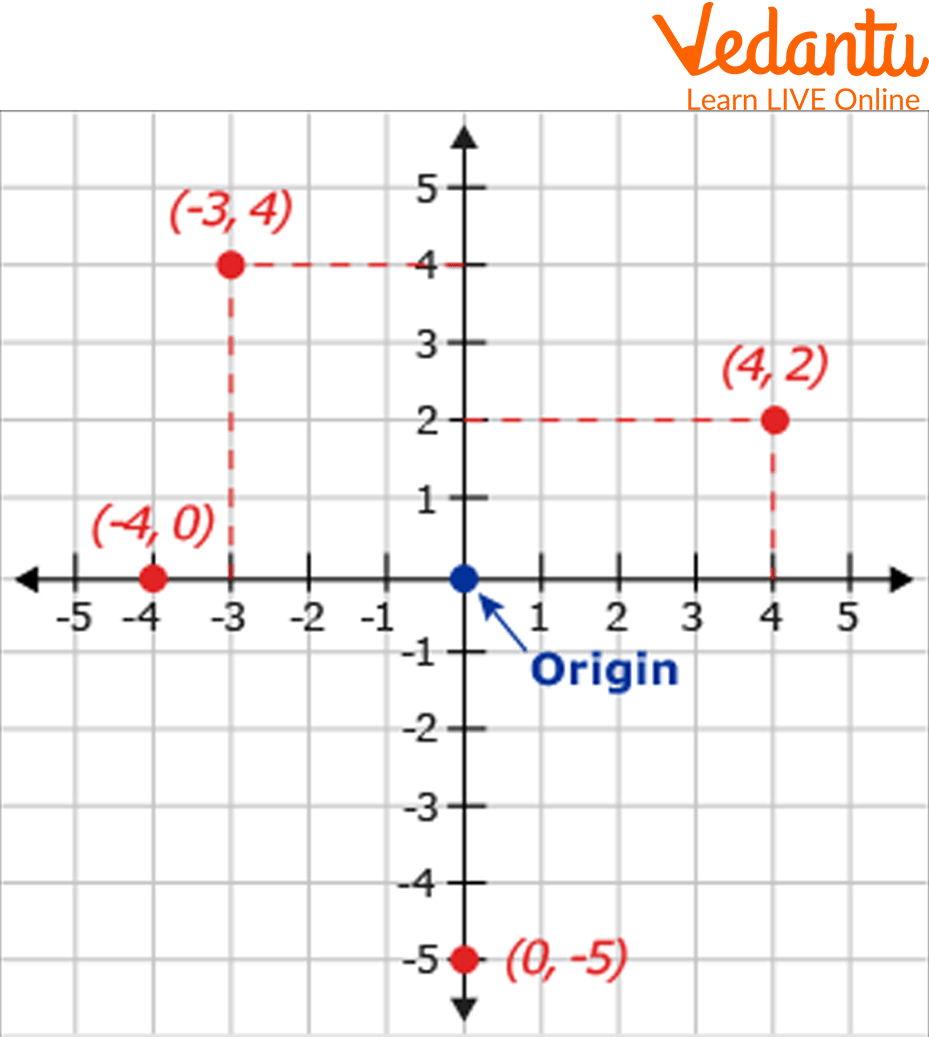Coordinates Images

## Practice Problems

Q1. Locate (5,6) on the coordinate graph.

Q2. Plot (-3.5,-2) on the XY coordinate graph.

Q3. Locate (2,-3) on the coordinate grid.

Q4. Show (-3,7) on the grid for coordinates.

## Summary

Summing up here with the concept of coordinates. This article covers the topics of grid for coordinates, quadrants, and x and y coordinates using a unique way that will have the students in gaining better. Here we have discussed in depth how to write the coordinates and how to locate them on the xy coordinate graph. Some practice problems along with solved examples have been shared so that students can practise well and gain proficiency in the concept deeply.

Last updated date: 23rd Sep 2023
Total views: 88.8k
Views today: 0.88k

## FAQs on What are Coordinates?

Q1. What are the x and y coordinates called?

The x coordinate is called the abscissa and the y coordinate is called an ordinate.

Q2. What is coordinate geometry?

Coordinate geometry is the branch of Mathematics in which we study geometrical figures by plotting them on the coordinate axes.

Q3. What do you mean by a graph?

Graph is a mathematical articulation of a network. It is generally used to describe the relationship between two things or objects. There are various types of graphs including bar graphs, histograms, pie charts, frequency polygons, etc.